Length of a hollow tube is 5m, it’s outer diameter is 10 cm and thickness of it’s wall is 5 mm. If the resistivity of the material of the tube is 1.7 × 10–8 Ω×m then the resistance of the tube will be :

(1) 5.6 × 10–5 Ω

(2) 2 × 10–5 Ω

(3) 4 × 10–5 Ω

(4) None of these

Concept Questions :-

Derivation of Ohm's law
High Yielding Test Series + Question Bank - NEET 2020

Difficulty Level:

A wire of resistor R is bent into a circular ring of radius r. Equivalent resistance between two points X and Y on its circumference, when angle XOY is α, can be given by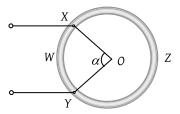(1) $\frac{R\alpha }{4{\pi }^{2}}\left(2\pi -\alpha \right)$

(2) $\frac{R}{2\pi }\left(2\pi -\alpha \right)$

(3) R (2πα)

(4) $\frac{4\pi }{R\alpha }\left(2\pi -\alpha \right)$

Concept Questions :-

Combination of resistors
High Yielding Test Series + Question Bank - NEET 2020

Difficulty Level:

The potential difference across the terminals of the battery shown in the figure is (r = internal resistance of battery) :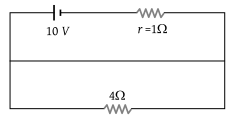(1) 8 V

(2) 10 V

(3) 6 V

(4) Zero

Concept Questions :-

Kirchoff's voltage law
High Yielding Test Series + Question Bank - NEET 2020

Difficulty Level:

As the switch S is closed in the circuit shown in the figure, the current passed through it is :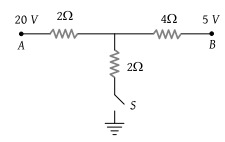(1) 4.5 A

(2) 6.0 A

(3) 3.0 A

(4) Zero

Concept Questions :-

Kirchoff's voltage law
High Yielding Test Series + Question Bank - NEET 2020

Difficulty Level:

In the following circuit, a 10 m long potentiometer wire with resistance 1.2 ohm/m, a resistance R1 and an accumulator of emf 2 V are connected in series. When the emf of the thermocouple is 2.4 mV then the deflection in the galvanometer is zero. The current supplied by the accumulator will be :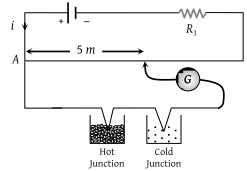(1) 4 × 10–4 A

(2) 8 × 10–4 A

(3) 4 × 10–3 A

(4) 8 × 10–3 A

Concept Questions :-

Meter bridge and potentiometer
High Yielding Test Series + Question Bank - NEET 2020

Difficulty Level:

In the following circuit, bulb rated as 1.5 V, 0.45 W. If bulbs glows with full intensity then what will be the equivalent resistance between X and Y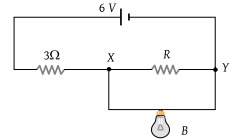(1) 0.45 Ω

(2) 1 Ω

(3) 3 Ω

(4) 5 Ω

High Yielding Test Series + Question Bank - NEET 2020

Difficulty Level:

Consider the circuits shown in the figure. Both the circuits are taking same current from battery but current through R in the second circuit is $\frac{1}{10}$th of current through R in the first circuit. If R is 11 Ω, the value of R1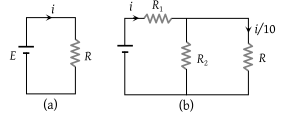(1) 9.9 Ω

(2) 11 Ω

(3) 8.8 Ω

(4) 7.7 Ω

Concept Questions :-

Combination of resistors
High Yielding Test Series + Question Bank - NEET 2020

Difficulty Level:

In order to quadruple the resistance of a uniform wire, a part of its length was uniformly stretched till the final length of the entire wire was 1.5 times the original length, the part of the wire was fraction equal to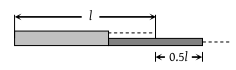(1) 1 / 8

(2) 1 / 6

(3) 1 / 10

(4) 1 / 4

Concept Questions :-

Combination of resistors
High Yielding Test Series + Question Bank - NEET 2020

Difficulty Level:

In the circuit shown in figure reading of voltmeter is V1 when only S1 is closed, reading of voltmeter is V2 when only S2 is closed and reading of voltmeter is V3 when both S1 and S2 are closed. Then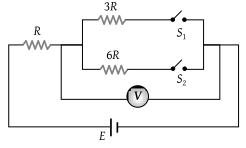(1) V3 > V2 > V1

(2) V2 > V1 > V3

(3) V3 > V1 > V2

(4) V1 > V2 > V3

Concept Questions :-

Combination of resistors
High Yielding Test Series + Question Bank - NEET 2020

Difficulty Level:

Current through wire XY of circuit shown is :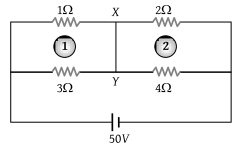(1) 1 A

(2) 4 A

(3) 2 A

(4) 3 A

Concept Questions :-

Combination of resistors